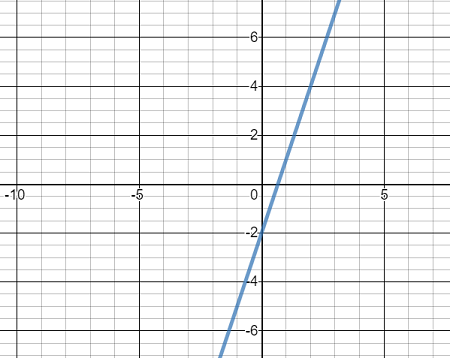# Draw the graph of the following function f(x)=3x-2

## Question:

Draw the graph of the following function {eq}f(x)=3x-2 {/eq}

## Linear Equation:

A linear equation is a type of equation which gives a straight line when plotted on a graph. The general form of a linear equation is,

{eq}\displaystyle { y = mx + c } {/eq}

where,

'm' is the slope and,

'c' is the y-intercept.

Let,

{eq}\displaystyle { y = f(x)=3x-2 } {/eq}

On comparing this equation with the general form of line equation we get,

{eq}\displaystyle { \text{Slope,} \ m = 3 \ \text{and} \\ \text{y-intercept}, \ c = -2 } {/eq}

Hence, the graph of this linear equation is,